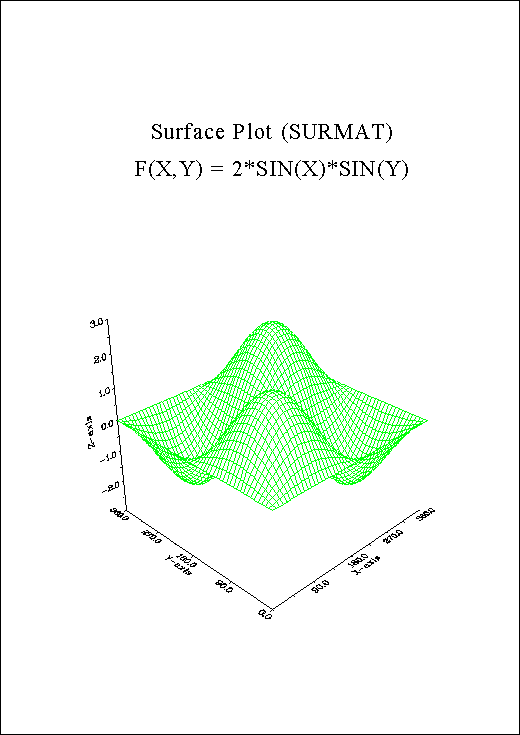## B.3 Surface Plot

```%GCL
// Surface Plot
N = 100
FLOAT ZMAT[N,N], XRAY[N]

PI = 3.1415927
STEP = 2. * PI / (N - 1)

DO I = 0, N - 1
XRAY[I] = SIN (I * STEP)
END DO

DO J = 0, N - 1
ZMAT[*,J] = 2 * XRAY * SIN (J * STEP)
END DO

METAFL ('CONS')
SETPAG ('DA4P')
DISINI ()
COMPLX ()
PAGERA ()

AXSPOS (200, 2600)
AXSLEN (1800, 1800)

NAME   ('X-axis', 'X')
NAME   ('Y-axis', 'Y')
NAME   ('Z-axis', 'Z')

TITLIN ('Surface plot (SURMAT)', 2)
TITLIN ('F(X,Y) = 2 * SIN(X) * SIN(Y)', 4)

VIEW3D (-5.,-5.,4.,'ABS')
GRAF3D (0.,360.,0.,90.,0.,360.,0.,90.,-3.,3.,-3.,1.)
HEIGHT (50)
TITLE  ()

COLOR  ('GREEN')
SHLSUR ()
SURMAT (ZMAT, N, N, 1, 1)

DISFIN ()
```Figure B.3: Surface Plot

Next | Previous | Contents